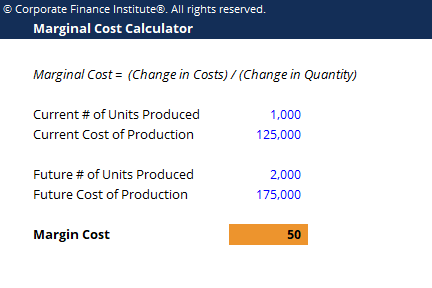# Marginal Cost Calculator

## Marginal Cost Calculator

This marginal cost calculator allows you to calculate the additional cost of producing more units using the formula:

Marginal Cost = Change in Costs / Change in Quantity

Here is a preview of the marginal cost calculator:### Marginal Cost Calculator

Marginal cost represents the incremental costs incurred when producing additional units of a good or service. It is calculated by taking the total change in costs of producing more goods and dividing that by the change in the quantity of good produced. The usual variable costs included in the calculation are labor and materials, plus the estimated increases in fixed costs (if any), such as administration, overhead, and selling expenses. The marginal cost formula can be used in financial modeling to optimize the generation of cash flow.

### More Free Templates

For more resources, check out our business templates library to download numerous free Excel modeling, PowerPoint presentation and Word document templates.

• Excel Modeling Templates
• PowerPoint Presentation Templates
• Transaction Document Templates

### Financial Analyst Certification

Become a certified Financial Modeling and Valuation Analyst (FMVA)® by completing CFI’s online financial modeling classes and training program!